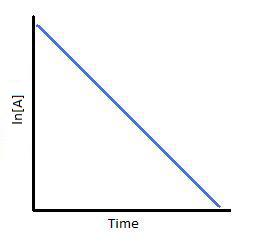Click to Chat

1800-1023-196

+91-120-4616500

CART 0

• 0

MY CART (5)

Use Coupon: CART20 and get 20% off on all online Study Material

ITEM
DETAILS
MRP
DISCOUNT
FINAL PRICE
Total Price: Rs.

There are no items in this cart.
Continue Shopping
`        What is ans. Of ques. 5a    and 6 plese see in the attatchment snd repy me`
one year ago

```							Dear Dushyant,The image you’ve provided is not clear, please attach a closer & a clearer image in order for me to decipher the questions!I’ll be happy to help you!regards,Soumendu
```
one year ago
```							5a) rate= -d[R]/dt=k[R]¹   ___ __  __ __ for first order reaction.Wher, [R]= concentration of reactants.d[R]/dt=-k[R]d[R]/[R]= -kdt∫ both sides∫d[R]/R=∫-kdt ln[R]= –Kt+C. ______________1When, t=0, R=[Ro]{initial concentration}ln[Ro]= CTherefore, 1=ln[R]=-Kt+ ln[Ro]kt=ln[Ro]-ln[R]k=ln[Ro/R]/tk=2.303log[Ro/R]/t viz the required equation.
```
one year ago
```							Dear Dushyant,Question-5:a) Let us use the following chemical equation: A ---> products.The decrease in the concentration of A over time can be written as: - d[A] / dt = k [A]Rearrangement yields the following: d[A] / [A] = - k dtIntegrate the equation, which yields: ln [A] = - kt + CEvaluate the value of C (the constant of integration) by using boundry conditions. Specifically, when t = 0, [A] = [A]o. [A]o is the original starting concentration of A.Substituting into the equation, we obtain: ln [A]o = - k (0) + C.Therefore, C = ln [A]oWe now can write the integrated form for first-order kinetics, as follows:ln [A] = - kt + ln [A]oThis last equation can be rearranged into several formats, such as:ln ([A] / [A]o) = - kt[A] / [A]o = [A] = [A]oQuestion-6:a) If [A]0 be initial concentration and k be rate constant then using above formula[A] = [A]o  = 0.8  {For 20% completion, concentration of reactants is [A]0 – (0.2)[A]0} (You can round off the value as per your convenience)Hence for 75% completionSo time taken for 75% completion is 62.126 minutes(approx).Question-6:b) t1/2 is called half-life of the radioactive substance which is the time taken for its concentration to become half of its initial concentration.Using above formula you will gett1/2 = Also for 99.9% completion time taken ist = Hence their ratio is given by (or relation between them is)Hope it helps!regards,Soumendu
```
one year ago
Think You Can Provide A Better Answer ?

## Other Related Questions on Physical Chemistry

View all Questions »### Course Features

• 731 Video Lectures
• Revision Notes
• Previous Year Papers
• Mind Map
• Study Planner
• NCERT Solutions
• Discussion Forum
• Test paper with Video Solution### Course Features

• 141 Video Lectures
• Revision Notes
• Test paper with Video Solution
• Mind Map
• Study Planner
• NCERT Solutions
• Discussion Forum
• Previous Year Exam Questions